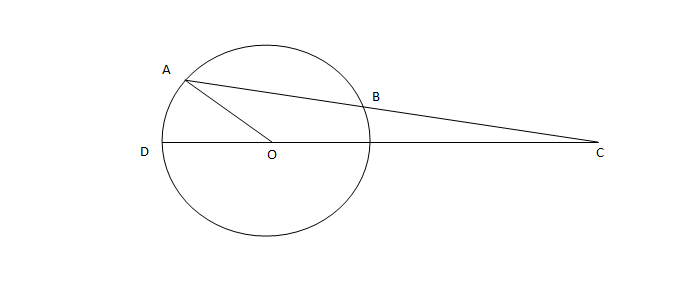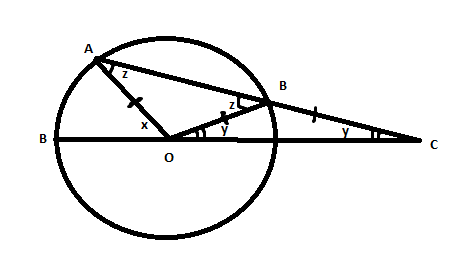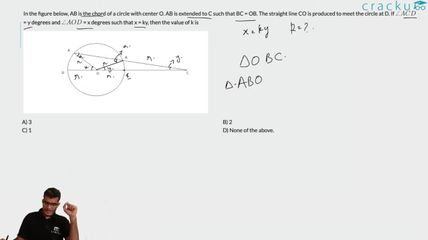Question 88

# In the figure below, AB is the chord of a circle with center O. AB is extended to C such that BC = OB. The straight line CO is produced to meet the circle at D. If $$\angle{ACD}$$ = y degrees and $$\angle{AOD}$$ = x degrees such that x = ky, then the value of k isSolutionSince Angle BOC = Angle BCO = y.

Angle OBC = 180-2y .

Hence Angle ABO =  z = 2y = Angle OAB.

Now since x is exterior angle of triangle AOC .

We have x = z + y = 3y.

Hence option A.

### View Video Solution